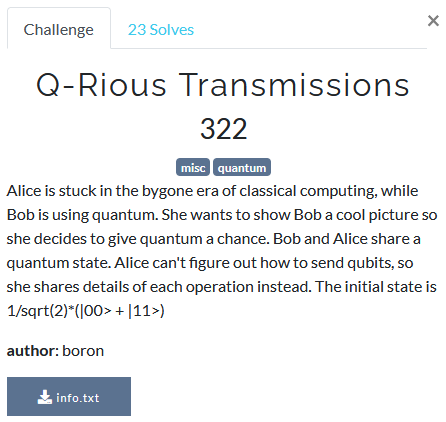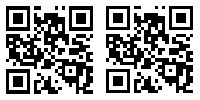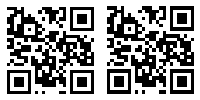# UIUCTF 2021 - Q-Rious Transmissions

Misc – 322 pts (23 solves) – Chall author: boron

Alice and Bob have shared two entangled qubits, which they use to transfer data between them using what appears to be similar to superdense coding (but without sending any qubits). Given only Alice’s operations on her own qubit, can we reconstruct what she send to Bob? Can we, or can we not? You won’t know until you collapse the state of this write-up. ;)

Check out write-ups by my teammates on K3RN3L4RMY.com

## ExplorationQuantum jokes aside, we are only given a file containing a sequence of elements in {I, X, Z, ZX}. Knowing that we are dealing with some cryptic quantum business these clearly refer to the respective quantum gates. Usually, when wanting to send information using qubits, Alice and Bob would make use of what is known as Superdense Coding. However, this makes use of two entangled qubits and requires Alice to send qubits to Bob. This is not the case here. Alice was most likely just alone in her room playing with her own qubit, applying the given sequence of quantum gates.

In order to get a better understanding of what was going on, let us consider how each of the quantum gates operate on a single qubit. I will use the [1,0] vector notation for a |0> qubit, and the [0,1] vector notation for a |1> qubit. In quantum theory, we have the possibility of having intermediate states, like |0> + |1> or |0> - |1>, however as you will see these do not occur using just the given sequence of quantum gates. Therefore I will not spend any time on that. Open the quantum gates!

$I \begin{bmatrix} a \\ b \end{bmatrix} = \begin{bmatrix} 1 & 0 \\ 0 & 1 \end{bmatrix} \begin{bmatrix} a \\ b \end{bmatrix} = \begin{bmatrix} a \\ b \end{bmatrix}$ $X \begin{bmatrix} a \\ b \end{bmatrix} = \begin{bmatrix} 0 & 1 \\ 1 & 0 \end{bmatrix} \begin{bmatrix} a \\ b \end{bmatrix} = \begin{bmatrix} b \\ a \end{bmatrix}$ $Z \begin{bmatrix} a \\ b \end{bmatrix} = \begin{bmatrix} 1 & 0 \\ 0 & -1 \end{bmatrix} \begin{bmatrix} a \\ b \end{bmatrix} = \begin{bmatrix} a \\ -b \end{bmatrix}$ $ZX \begin{bmatrix} a \\ b \end{bmatrix} = \begin{bmatrix} 1 & 0 \\ 0 & -1 \end{bmatrix} \begin{bmatrix} 0 & 1 \\ 1 & 0 \end{bmatrix} = \begin{bmatrix} 0 & 1 \\ -1 & 0 \end{bmatrix} \begin{bmatrix} a \\ b \end{bmatrix} = \begin{bmatrix} b \\ -a \end{bmatrix}$

Assuming Alice starts with either a [1,0] or [0,1] state qubit, there is no way to turn it into intermediate states, so a measurement of her qubit will always yield 0 or 1 deterministically. So we just apply each gate in the sequence, measure the qubit, and move on. Furthermore, there is no measurable difference between [1,0] and [-1,0], they both return a ‘0’ bit. Therefore the effect of the gates boils down to:

• $I$, nothing
• $X$, flip the bit
• $Z$, nothing
• $ZX$, flip the bit

Seems to me we can just parse the gate sequence without modelling any qubits.

## Exploitation

If we start with a ‘0’ bit and simply add the last bit of the list after every gate, only flipping the bit upon reading a X or ZX gate, we can retrieve the data very easily. By looking at the bitstream that comes out one should easily notice it forms an image and not proper ASCII.

bits = 

for i in sequence:
if i in ['X', 'ZX']:
bits += [(bits[-1] + 1) % 2]
else:
bits += [bits[-1]]

bits = bits[1:]

im0 = Image.new('1',(200,100))
im0.putdata(bits)
display(im0)And there we go. Not much quantum about that, hah. Hand over that physics degree, professor!

I was a bit disappointed though to find out the challenge turned out to be quite trivial. I was excited to use QisKit and so I did it anyway. Below you find a script simulating a single qubit where we apply all sequence gates exactly as provided and measure the state of the qubit after every gate. Go, go, qubit!

#!/usr/bin/env python3
#
# Polymero
#

# Imports
from qiskit import QuantumCircuit, Aer, assemble
from PIL import Image

print("Reading in Alice's file...")

# Read in Alice's operation sequence
sequence = data.split()
f.close()

def do_op( qc, op ):
""" Applies one of the {I, Z, X, ZX} gates to an input qubit """
if op == 'ZX':
qc.z(0)
qc.x(0)
elif op == 'X':
qc.x(0)
elif op == 'Z':
qc.z(0)
elif op == 'I':
pass
else:
print('Operation not recognised:', op)

print("Applying Alice's operations...")

# Make a QuantumCircuit with a single qubit
qc = QuantumCircuit(1,len(sequence))
for i in range(len(sequence)):
# Apply Alice's operation
do_op(qc, sequence[i])
# Measure the resulting state of the qubit
qc.measure(0,i)

print('Running the simulation...')

# Tell Qiskit which simulator to use
sim = Aer.get_backend('aer_simulator')
# Create a Qobj with all our circuits for the simulator
qobj = assemble(qc)
# Simulate each circuit only once (determinisitc)
result = sim.run(qobj, shots=1).result()

print('Done!')

# Convert simulation result to bit stream
bitstream = [int(i) for i in list(list(result.get_counts()))]

# Convert bitstream to an image
im0 = Image.new('1',(200,100))
im0.putdata(bitstream)
display(im0)Seems like that worked as well. Nice! Only cool kids use QisKit, hehe.

Ta-da!

uiuctf{5up3rqu4ntum_1m4g3ry}